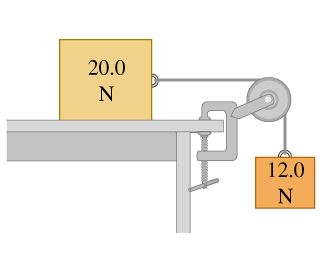# Problem: Two blocks are connected by a very light string passing over a massless and frictionless pulley (the figure ). Traveling at constant speed, the 20.0-N block moves 76.0 cm to the right and the 12.0-N block moves 76.0 cm downward.During this process, how much work is done on the 12.0-N block by the tension in the string?During this process, how much work is done on the 20.0-N block by the normal force?Find the total work done on 12.0-N block.During this process, how much work is done on the 12.0-N block by gravity?During this process, how much work is done on the 20.0-N block by gravity?During this process, how much work is done on the 20.0-N block by the tension in the string?During this process, how much work is done on the 20.0-N block by friction?Find the total work done on 20.0-N block.

###### FREE Expert Solution
88% (409 ratings)
###### Problem Details

Two blocks are connected by a very light string passing over a massless and frictionless pulley (the figure). Traveling at constant speed, the 20.0-N block moves 76.0 cm to the right and the 12.0-N block moves 76.0 cm downward.

During this process, how much work is done on the 12.0-N block by the tension in the string?

During this process, how much work is done on the 20.0-N block by the normal force?

Find the total work done on 12.0-N block.

During this process, how much work is done on the 12.0-N block by gravity?

During this process, how much work is done on the 20.0-N block by gravity?

During this process, how much work is done on the 20.0-N block by the tension in the string?

During this process, how much work is done on the 20.0-N block by friction?

Find the total work done on 20.0-N block.